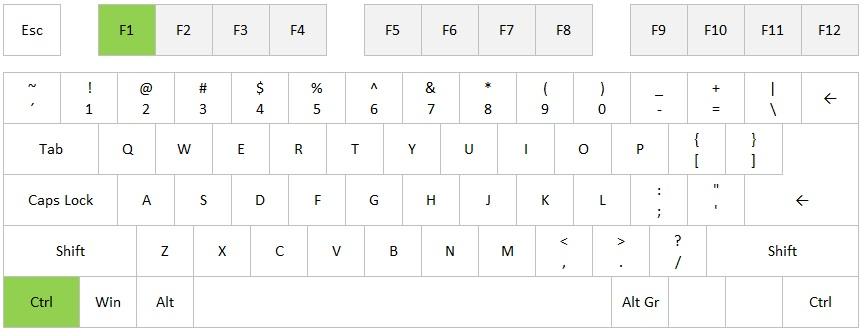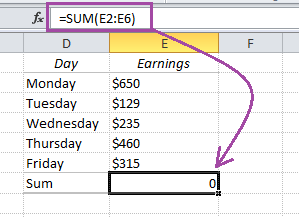# Summarize a whole table in Excel without writing a formula

Instead of typing a SUM formula, an easier way to go is to use the shortcut Alt + Equal sign (=)

But did you know that you can use this shortcut for a whole table?

If you have a table like the one below, select all the values plus one extra row and one extra column:

# How to handle parts per million, basis points and per mille in Excel

Excel has built-in functionality to handle percent (%), but there is no automatic way to calculate parts per million, basis points, permyriad or per mille in Excel. It is easy to calculate though, but let’s start with the definitions:

And here’s how to apply it:

# Hide or show Ribbon in Excel

The ribbon in Excel, which was introduced in Excel 2007, is a major improvement from the old menus. But it takes up a lot of space, so sometimes you want to hide it. There are several ways to do it, but all you have to learn is the shortcut:

Hide ribbon: Ctrl + F1

Show ribbon: Ctrl + F1This also works in Word, Powerpoint and Outlook 2007 and later

Are you using a non-English version of Excel? Click here for translations of the 100 most common functions.

# Convert text to numbers in Excel using formulasHave you ever imported data into Excel that won’t calculate? Look at this example where the earnings look like numbers to the human eye, but not to Excel. And when Excel is not able to identify any numbers, it can’t calculate any sums or averages either.

This happens all the time if you use data from other data sources: It could be a txt-file from a booking system, a copy-paste from a website or even a database export.

Enough about the problem. Let’s solve it, and let’s not spend more than 2 minutes doing it!

# Yellow pop-up box when selecting cell in Excel

Wouldn’t it be cool if you could have a yellow info note that pops up when you select the cell in Excel? Actually you can! Let’s look at a little used feature of Data Validation.

It’s easy and you can do it in less than a minute!